4.9.3 Better Iterative Traversal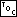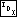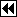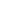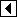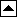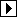We have developed an efficient, convenient function for traversing a binary tree. In the exercises, we made it reliable, and it is possible to make it resilient as well. But its algorithm makes it difficult to add generality. In order to do that in a practical way, we will have to use a new algorithm.

The other half is the successor of a node that doesn't have a right child. Take a look at the code for one of the previous traversal functions—recursive or iterative, whichever you better understand—and mentally work out the relationship between the current node and its successor for a node without a right child. What happens is that we move up the tree, from a node to its parent, one node at a time, until it turns out that we moved up to the right (as opposed to up to the left) and that is the successor node. Think of it this way: if we move up to the left, then the node we started at has a lesser value than where we ended up, so we've already visited it, but if we move up to the right, then we're moving to a node with a greater value, so we've found the successor.

Using these instructions, we can find the predecessor of a node, too, just by exchanging “left” and “right”. This suggests that all we have to do in order to generalize our traversal function is to keep track of all the nodes above the current node, not just the ones that are up and to the left. This in turn suggests our final implementation of struct bst_traverser, with appropriate comments:

```61. <BST traverser structure 61> =
/* BST traverser structure. */
struct bst_traverser   {
struct bst_table *bst_table;        /* Tree being traversed. */
struct bst_node *bst_node;          /* Current node in tree. */
struct bst_node *bst_stack[BST_MAX_HEIGHT];                                         /* All the nodes above bst_node. */
size_t bst_height;                  /* Number of nodes in bst_parent. */
unsigned long bst_generation;       /* Generation number. */
};
```

This code is included in 24, 142, and 192.

Because user code is expected to declare actual instances of struct bst_traverser, struct bst_traverser must be defined in <bst.h 24> and therefore all of its member names are prefixed by bst_ for safety.

The only surprise in struct bst_traverser is member bst_generation, the traverser's generation number. This member is set equal to its namesake in struct bst_table when a traverser is initialized. After that, the two values are compared whenever the stack of parent pointers must be accessed. Any change in the tree that could disturb the action of a traverser will cause their generation numbers to differ, which in turn triggers an update to the stack. This is what allows this final implementation to be resilient.

We need a utility function to actually update the stack of parent pointers when differing generation numbers are detected. This is easy to write:

```62. <BST traverser refresher 62> =
/* Refreshes the stack of parent pointers in trav
and updates its generation number. */
static void trav_refresh (struct bst_traverser *trav) {
assert (trav != NULL);

trav->bst_generation = trav->bst_table->bst_generation;

if (trav->bst_node != NULL)     {
bst_comparison_func *cmp = trav->bst_table->bst_compare;
void *param = trav->bst_table->bst_param;
struct bst_node *node = trav->bst_node;
struct bst_node *i;

trav->bst_height = 0;
for (i = trav->bst_table->bst_root; i != node; )         {
assert (trav->bst_height < BST_MAX_HEIGHT);
assert (i != NULL);

trav->bst_stack[trav->bst_height++] = i;
i = i->bst_link[cmp (node->bst_data, i->bst_data, param) > 0];
}
}
}
```

This code is included in 63 and 178.

The following sections will implement all of the traverser functions bst_t_*(). See Traversers, for descriptions of the purpose of each of these functions.

The traversal functions are collected together into <BST traversal functions 63>:

```63. <BST traversal functions 63> =
<BST traverser refresher 62>
<BST traverser null initializer 64>
<BST traverser least-item initializer 65>
<BST traverser greatest-item initializer 66>
<BST traverser search initializer 67>
<BST traverser insertion initializer 68>
<BST traverser copy initializer 69>
<BST traverser advance function 70>
<BST traverser back up function 73>
<BST traverser current item function 74>
<BST traverser replacement function 75>
```

This code is included in 29.

Exercises:

1. The bst_probe() function doesn't change the tree's generation number. Why not? [answer]

*2. The main loop in trav_refresh() contains the assertion

```      assert (trav->bst_height < BST_MAX_HEIGHT);
```

Prove that this assertion is always true. [answer]

3. In trav_refresh(), it is tempting to avoid calls to the user-supplied comparison function by comparing the nodes on the stack to the current state of the tree; e.g., move up the stack, starting from the bottom, and for each node verify that it is a child of the previous one on the stack, falling back to the general algorithm at the first mismatch. Why won't this work? [answer]